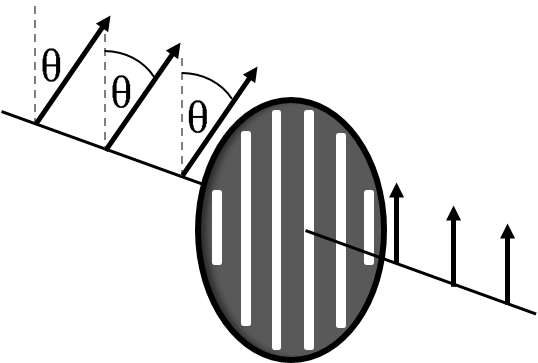# Malus Law Calculator

Created by Wojciech Sas, PhD candidate
Reviewed by Bogna Szyk
Last updated: May 18, 2020

Malus law calculator is a helpful tool which explains the functional principle of polarizers. Depending on the relative position between the direction of light polarization and the axis of a polarizer, the different amount of light can be transmitted. By knowing the initial intensity of light and the polarization orientation, you can easily find the output intensity, which comes out of the polarizer, based on Malus law.

## Light polarization

Light can be described as mutually oscillating electric and magnetic fields forming an electromagnetic wave. These oscillations are perpendicular to each other and simultaneously perpendicular to the direction of wave propagation.

The light is said to be linearly polarized if the orientation of oscillations is constant in time. It is common to say that the direction of light polarization is the same as the one of electric field oscillation. Polarized light can be obtained due to reflection on the boundary of two media with different refractive indexes if the angle of incidence equals Brewster's angle or by passing light through some dichroic crystal.

## How does a polarizer work?

Polarizers are usually made out of oblong shaped molecules, all aligned in the same direction. It turns out that if the polarization of the incident beam is the same as alignment orientation, then the light is most likely to be absorbed. If the polarization is perpendicular to the long axis of molecules, then it is transmitted almost entirely and that direction is the axis of the polarizer. If the angle of polarization is something in between, it passes through only partially and its initial irradiance decreases. The exact value can be determined thanks to the Malus law.## Malus law formula

Irradiance (intensity) of light is the amount of power received on the unit of surface, so it is usually measured in W/m². Considering the wavelengths of photons, it can also be converted into luxes, units of illuminance. The light intensity, which passes through the ideal polarizer can be calculated as:

intensity = initial_intensity * cos²θ,

where:

• initial_intensity is the irradiance of incident light,
• θ is the angle between the light polarization and the axis of the polarizer,
• intensity is the output irradiance.

## Irradiance changes under rotation - an example

Let's say that you want to check how the intensity of polarized light changes, while you rotate your polarizer.

1. Choose a few different values of the axis of polarizer orientation with respect to the polarization of incident rays, e.g. θ₁=20°, θ₂=45°, θ₃=70°,

2. Determine cosθ of this angles, which are 0.939, 0.707, 0.342 respectively,

3. Find squares of this values: 0.883, 0.5, 0.117,

4. Multiply them by the initial intensity, say I₀=5 W/m²: I₁=4.415 W/m², I₂=2.5 W/m², I₃=0.585 W/m²...

5. Or simply enter data to this Malus law calculator and save your time!

You can always express obtained results as the percentages of initial intensity.

## Polarizers in everyday life

Have you ever wondered why it is possible to remove light reflections on water surface only by rotating the polarizing filter in your camera? Now you know the secret and, what's more, you can easily predict the effect using Malus law calculator. Polarized sunglasses are also based on this phenomenon so that they are able to block a vast majority of polarized light during sunny days.

Wojciech Sas, PhD candidate
Initial Intensity
W/
Angle of incidence
deg
Intensity
W/
ft²
People also viewed…

### Buoyancy

Buoyancy calculator finds the buoyant force acting on an object immersed in water.

### Circle skirt

Circle skirt calculator makes sewing circle skirts a breeze.

### Heisenberg uncertainty

Learn about the Heisenberg uncertainty principle equation and the relationship between the uncertainty of position, momentum, and velocity in quantum mechanics.